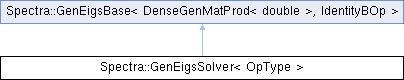Spectra  1.0.1 Header-only C++ Library for Large Scale Eigenvalue Problems
Spectra::GenEigsSolver< OpType > Class Template Reference

#include <Spectra/GenEigsSolver.h>

Inheritance diagram for Spectra::GenEigsSolver< OpType >:## Public Member Functions

GenEigsSolver (OpType &op, Index nev, Index ncv)Public Member Functions inherited from Spectra::GenEigsBase< DenseGenMatProd< double >, IdentityBOp >
void init (const Scalar *init_resid)

void init ()

Index compute (SortRule selection=SortRule::LargestMagn, Index maxit=1000, Scalar tol=1e-10, SortRule sorting=SortRule::LargestMagn)

CompInfo info () const

Index num_iterations () const

Index num_operations () const

ComplexVector eigenvalues () const

ComplexMatrix eigenvectors (Index nvec) const

ComplexMatrix eigenvectors () const

## Detailed Description

### template<typename OpType = DenseGenMatProd<double>> class Spectra::GenEigsSolver< OpType >

This class implements the eigen solver for general real matrices, i.e., to solve $$Ax=\lambda x$$ for a possibly non-symmetric $$A$$ matrix.

Most of the background information documented in the SymEigsSolver class also applies to the GenEigsSolver class here, except that the eigenvalues and eigenvectors of a general matrix can now be complex-valued.

Template Parameters
 OpType The name of the matrix operation class. Users could either use the wrapper classes such as DenseGenMatProd and SparseGenMatProd, or define their own that implements the type definition Scalar and all the public member functions as in DenseGenMatProd.

An example that illustrates the usage of GenEigsSolver is give below:

#include <Eigen/Core>
#include <Spectra/GenEigsSolver.h>
// <Spectra/MatOp/DenseGenMatProd.h> is implicitly included
#include <iostream>
using namespace Spectra;
int main()
{
// We are going to calculate the eigenvalues of M
Eigen::MatrixXd M = Eigen::MatrixXd::Random(10, 10);
// Construct matrix operation object using the wrapper class
DenseGenMatProd<double> op(M);
// Construct eigen solver object, requesting the largest
// (in magnitude, or norm) three eigenvalues
GenEigsSolver<DenseGenMatProd<double>> eigs(op, 3, 6);
// Initialize and compute
eigs.init();
int nconv = eigs.compute(SortRule::LargestMagn);
// Retrieve results
Eigen::VectorXcd evalues;
if (eigs.info() == CompInfo::Successful)
evalues = eigs.eigenvalues();
std::cout << "Eigenvalues found:\n" << evalues << std::endl;
return 0;
}
@ Successful
Computation was successful.

And also an example for sparse matrices:

#include <Eigen/Core>
#include <Eigen/SparseCore>
#include <Spectra/GenEigsSolver.h>
#include <Spectra/MatOp/SparseGenMatProd.h>
#include <iostream>
using namespace Spectra;
int main()
{
// A band matrix with 1 on the main diagonal, 2 on the below-main subdiagonal,
// and 3 on the above-main subdiagonal
const int n = 10;
Eigen::SparseMatrix<double> M(n, n);
M.reserve(Eigen::VectorXi::Constant(n, 3));
for (int i = 0; i < n; i++)
{
M.insert(i, i) = 1.0;
if (i > 0)
M.insert(i - 1, i) = 3.0;
if (i < n - 1)
M.insert(i + 1, i) = 2.0;
}
// Construct matrix operation object using the wrapper class SparseGenMatProd
SparseGenMatProd<double> op(M);
// Construct eigen solver object, requesting the largest three eigenvalues
GenEigsSolver<SparseGenMatProd<double>> eigs(op, 3, 6);
// Initialize and compute
eigs.init();
int nconv = eigs.compute(SortRule::LargestMagn);
// Retrieve results
Eigen::VectorXcd evalues;
if (eigs.info() == CompInfo::Successful)
evalues = eigs.eigenvalues();
std::cout << "Eigenvalues found:\n" << evalues << std::endl;
return 0;
}

Definition at line 119 of file GenEigsSolver.h.

## ◆ GenEigsSolver()

template<typename OpType = DenseGenMatProd<double>>
 Spectra::GenEigsSolver< OpType >::GenEigsSolver ( OpType & op, Index nev, Index ncv )
inline

Constructor to create a solver object.

Parameters
 op The matrix operation object that implements the matrix-vector multiplication operation of $$A$$: calculating $$Av$$ for any vector $$v$$. Users could either create the object from the wrapper class such as DenseGenMatProd, or define their own that implements all the public members as in DenseGenMatProd. nev Number of eigenvalues requested. This should satisfy $$1\le nev \le n-2$$, where $$n$$ is the size of matrix. ncv Parameter that controls the convergence speed of the algorithm. Typically a larger ncv means faster convergence, but it may also result in greater memory use and more matrix operations in each iteration. This parameter must satisfy $$nev+2 \le ncv \le n$$, and is advised to take $$ncv \ge 2\cdot nev + 1$$.

Definition at line 142 of file GenEigsSolver.h.

The documentation for this class was generated from the following file: